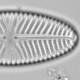## Comparison of reconstruction diagnostics

Over the previous few posts, I have been demonstrating diagnostics for reconstructions based on individual fossil assemblages. I promised to compare the four diagnostics I have presented, but first I need to demonstrate a fifth which I forgot earlier.

Reconstructions based on a bootstrap sample of the calibration set, a random sample with replacement the same size as the original calibration set so some observations are selected several times and others not at all, will differ from reconstructions based on another bootstrap sample. The amount of variation between reconstructions based on many bootstrap samples can be used as a diagnostic of the reliability of the reconstruction. The more variable the reconstruction is, the more more sensitive it is to small changes in the calibration set. The standard deviation of the bootstrap reconstructions is used.

Unlike all the other assemblage-based diagnostics I have presented, this diagnostic is dependent on the transfer function method being used. With weighted average (WA) based methods, the reconstructions vary between bootstraps because of uncertainty in the species’ optima, especially the taxa with few effective occurrences. With the modern analogue technique (MAT), the reconstructions vary between bootstrap samples as different analogues are available for selection, so if possible analogues have different environmental conditions, the bootstrap variability will be high.

I’m going to show how the standard deviation of bootstrap reconstructions can be calculated with the `rioja` package. First I load the data.

```library(rioja)
data(SWAP, RLGH)
spp<-SWAP\$spec
env<-SWAP\$pH
fos<-RLGH\$spec
age<-RLGH\$depths\$Age
```

Now I fit a WA and a MAT model to the calibration set data. With MAT I’ve asked for five analogues and asked it to retain the distances with the argument `lean=FALSE` (`predict( )` will complain if you don’t do this). Then I make predictions with the predict function. I ask for the uncertainties to be calculated with the argument `sse=TRUE` and ask for 1000 bootstrap samples (more is better but slower).

```modWA<-WA(spp, env)
modMAT<-MAT(spp, env, k=5, lean=FALSE)

predWA<-predict(modWA, newdata=fos, sse=TRUE, nboot=1000)
predMAT<-predict(modMAT, newdata=fos, sse=TRUE, nboot=1000)
names(predWA)

x11(height=5,4.5);par(mfrow=c(2,1), mar=c(3,3,1,1), mgp=c(1.5,.5,0))
plot(age, predWA\$v1.boot[,1], ylab="bootstrap sd")
plot(age, predMAT\$v1.boot[,1], ylab="bootstrap sd",col=2, pch=2)
```Standard deviation of the WA (upper) and MAT (lower) reconstructions from the Round Loch of Glenhead.

Both the pattern and absolute value of the standard deviation of the bootstrap residuals differ greatly between the two models. This is not greatly surprising as they are dependent on different aspects of the data. The MAT sd is almost half the RMSEP, whereas the WA sd is much smaller as a proportion of its RMSEP. MAT may be struggling to find good analogues in this relatively small calibration set.

I now want to compare the different diagnostics that I have developed – do they show similar patterns or are they capturing different aspects. I’m simply going to present a `pairs( )` plot and a correlation matrix of the different diagnostics for the Round Loch of Glenhead diatom stratigraphy.

Some of these diagnostics are numerically independent of each other, for example the proportion of the fossil assemblage that is missing from the calibration set is numerically independent of the proportion of species with poorly defined optima, residual length (`residLen` uses a left join to omit the missing taxa), and the standard deviation of the WA reconstructions. The missing taxa should however contribute to the analogue distance. The proportion of species with poorly defined optima should influence the standard deviation of the WA reconstructions as the latter depends on the uncertainty in the optima.

This function runs the all the different diagnostics and returns the result as a data.frame.

```all.diagnostics<-function(spp, env, fos){
require(rioja)
allspp<-Merge(spp, fos, split=TRUE)
spp2<-allspp\$spp
fos2<-allspp\$fos
n2<-Hill.N2(spp2)

modmat<-MAT(spp/100, env,k=5, lean=FALSE)
predmat<-predict(modmat, fos/100, sse=T, nboot=1000)
modwa<-WA(spp, env)
predwa<-predict(modwa, fos, sse=T, nboot=1000)
res<-list()
res\$residlen<-residLen(spp, env, fos, method="cca")\$passive#residual length
res\$sum0<-apply(fos2,1,function(x)sum(x[is.infinite(n2)]))#missing from calibration set
res\$sum5<-apply(fos2,1,function(x)sum(x[n2<=5]))#poorly defined optima
res\$analogue<-predmat\$dist.n[,1]#distance to nearest analogue
res\$S1mat<-predmat\$v1.boot[,1]#standard deviation of MAT bootstrap estimates
res\$S1wa<-predwa\$v1.boot[,1] #standard deviation of WA bootstrap estimates
as.data.frame(res)
}
```

With the SWAP and RLGH data

```rlghres<-all.diagnostics(spp, env, fos)
pairs(rlghres, gap=0)
round(cor(rlghres), 2)
#        residlen  sum0  sum5 analogue S1mat  S1wa
#residlen     1.00 -0.39  0.34    -0.12  0.39 -0.10
#sum0        -0.39  1.00 -0.15     0.06 -0.44  0.32
#sum5         0.34 -0.15  1.00     0.37  0.29 -0.37
#analogue    -0.12  0.06  0.37     1.00 -0.24 -0.03
#S1mat        0.39 -0.44  0.29    -0.24  1.00 -0.44
#S1wa        -0.10  0.32 -0.37    -0.03 -0.44  1.00
```Pairs plot of different diagnostics for the Round Loch of Glenhead reconstructions.

The different reconstruction diagnostics are rather weakly correlated; they appear to share rather little information. This might be because the RLGH data are well behaved and have no assemblages with many missing species or large analogue distances.
If the result presented here represents the general case then it would suggest that we should check all the diagnostics for problems and report any issues.

Which is the most important diagnostic to report or plot in a paper? The worst! If a large proportion of the assemblage is missing from the calibration set, this should be reported. If many assemblages lack good analogues, that should be reported. If all are bad, it might be sensible not to publish the reconstruction!

I need to look at more fossil stratigraphies and calibration sets to determine whether the patterns found here are general. If the results are interesting, it might just make a paper rather than a blog post.